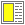# Exercise 2.9 Colors in HTML **

In HTML we define colors as text strings of length 7:

`    "#rstuvw"`

The symbols r, s, t, u, v, and w are all hexadecimal numbers between 0 and f (15). rs is in that way the hexadecimal representation for red, tu is the code for green, and vw is the code for blue.

As an example, the text string

`    "#ffffff"`

represents white and

`    "#ff0000"`

is red.

In Scheme we wish to represent a color as the list

`    (color r g b)`

where color is a symbol, r is number between 0 and 255 which represents the amount of red, and g and b in a similar way the amount of green and blue in the color.

Write a Scheme function that transforms a Scheme color to a HTML color string.

It is a good training to program the function that converts decimal numbers to hexa decimal numbers. I suggest that you do that - I did it in fact in my solution! If you want to make life a little easier, the Scheme function (number->string n radix) is helpful (pass radix 16 as second parameter).